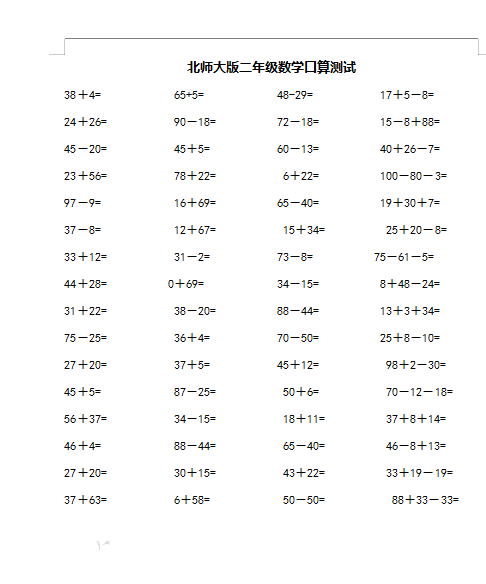38＋4=            65+5=            48-29=           17＋5－8=

24＋26=           90－18=          72－18=          15－8＋88=

45－20=           45＋5=           60－13=          40＋26－7=

23＋56=           78＋22=           6＋22=          100－80－3=

97－9=            16＋69=          65－40=          19＋30＋7=

37－8=            12＋67=           15＋34=          25＋20－8=

2022：新北师大版小学数学2年级下册口算 (8)（doc文档2电页子档下载）